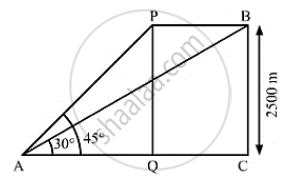# The Angle of Elevation of an Aeroplane from a Point on the Ground is 45° After Flying for 15seconds, the Elevation Changes to 30° . Find the Speed of the Areoplane. - Mathematics

The angle of elevation of an aeroplane from a point on the ground is 45° after flying for 15seconds, the elevation changes to 30° . If the aeroplane is flying at a height of 2500 meters, find the speed of the areoplane.

#### SolutionLet the height of flying of the aero-plane be PQ = BC and point A be the point of observation.
We have,
PQ = BC = 2500m, ∠PAQ = 45° and ∠BAC = 30°
In ΔPAQ,

 tan 45° = (PQ)/(AQ)

⇒ 1 = 2500/(AQ)

⇒AQ = 2500  m

Also, in ΔABC,

tan 30° = (BC)/(AC)

⇒1/ sqrt(3) = 2500/(AC)

⇒ AC = 2500 sqrt(3)  m

Now , QC = AC - AQ

= 2500 sqrt(3)-2500

= 2500( sqrt(3)-1)  m

= 2500 (1.732  - 1)

= 2500(0.732)

= 1830  m

⇒ PB = QC = 1830

So, the speed of the aero-plane(PB)/15

= 1830/15

=122 m/s

=122 xx3600/1000   km ⁄ h

= 439.2 km /5

So, the speed of the aero-plane is 122m/ s or 439.2 km/ h.

Concept: Heights and Distances
Is there an error in this question or solution?

#### APPEARS IN

RS Aggarwal Secondary School Class 10 Maths
Chapter 14 Height and Distance
Exercises | Q 25

Share